﻿ Notes on a Double Inequality for Ratios of any Two Neighbouring Non-zero Bernoulli NumbersPublications are Open
Access in this journal
Article Versions
Export Article
• Normal Style
• MLA Style
• APA Style
• Chicago Style
Research Article
Open Access Peer-reviewed

### Notes on a Double Inequality for Ratios of any Two Neighbouring Non-zero Bernoulli Numbers

Feng Qi
Turkish Journal of Analysis and Number Theory. 2018, 6(5), 129-131. DOI: 10.12691/tjant-6-5-1
Received June 17, 2018; Revised August 04, 2018; Accepted September 09, 2018

### Abstract

In the paper, the author notes on a double inequality published in “Feng Qi, A double inequality for the ratio of two non-zero neighbouring Bernoulli numbers, Journal of Computational and Applied Mathematics 351 (2019), 1-5; Available online at https://doi.org/10.1016/j.cam.2018.10.049.”

### 1. Introduction

We recall from [ 1, p. 804, 23.1.1] and [ 2, p. 3, (1.1)] that the Bernoulli numbers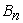can be generated by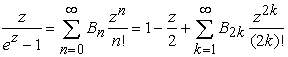for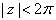. It is easy to verify that the function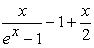is even in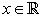. Consequently, all the Bernoulli numbers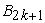for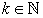equal 0.

To discover explicit formulas, recurrent formulas, closed expressions, and integral representations of the Bernoulli numbers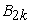foris a classical topic. For recently published results, please refer to the papers 3, 4, 5, 6, 7, 8, 9, 10, 11, 12 and closely related references therein.

To bound the Bernoulli numbersforby inequalities is an alternative topic. In [ 1, p. 805, 23.1.15], [ 13, Theorem 1.1], [ 2, p. 14, (1.23) and p. 23, Exercise 1.2], and the papers 14, 15, 16, some inequalities for bounding the Bernoulli numberswere established and collected. Most of these inequalities have been refined or sharpened in 17 by the double inequality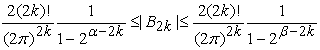(1)

for, where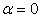and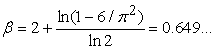are the best possible in the sense that they can not be replaced respectively by any bigger and smaller constants in the double inequality (1).

To study the differences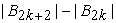and the ratios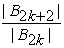foris also an interesting topic. In the newly published paper 18, the ratiosfor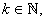which is equivalent to the differences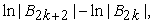were bounded by the double inequality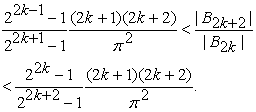(2)

Motivated by the double inequality (2) and by the fact that the function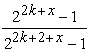is strictly increasing in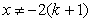for allwe naturally pose a problem: what are the best constants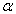and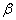such that the double inequality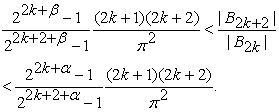(3)

is valid for all?

In [ 2, p. 5, (1.14)], it was listed that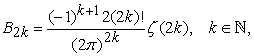where the Riemann zeta function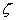can be defined 19, 20, 21 by the series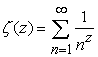under the condition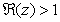and by analytic continuation elsewhere.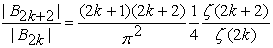(4)

for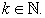By virtue of (4), the double inequality (3) can be rewritten as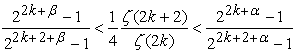(5)

which can be further reformulated as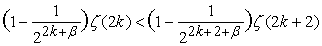and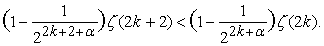Let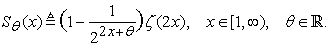Then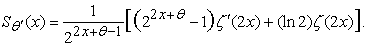In order that the function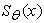is strictly increasing (or strictly decreasing, respectively) on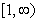, it is necessary and sufficient that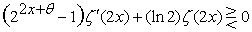on, which can be rearranged as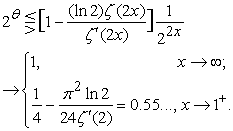Consequently, in order that the functionfor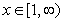and the sequence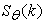withare strictly increasing (or strictly decreasing, respectively), it is necessary that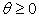(or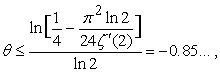respectively). The double inequality (5) can also be reformulated as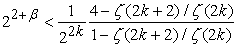and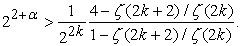Since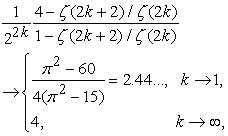It follows that the necessary conditions are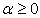and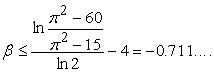This implies that the right-hand side inequality in (2) is sharp, but the left-hand side inequality in (2) perhaps can be improved. In conclusion, we guess that the double inequality (3) is valid if and only ifand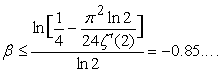Since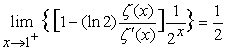and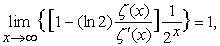we guess that the function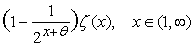is strictly increasing (or strictly decreasing, respectively) if and only if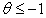(or, respectively).

The double inequality (2) has been cited and applied in the papers 22, 23, 24, 25, 26, 27, 28, 29.

Can one generalize the inequality (2) to the case for the Bernoulli polynomials?

This paper and 18 are respectively extracted from the preprints 30, 31, 32, 33.

### References

  M. Abramowitz and I. A. Stegun (Eds), Handbook of Mathematical Functions with Formulas, Graphs, and Mathematical Tables, National Bureau of Standards, Applied Mathematics Series 55, 10th printing, Washington, 1972. In article  N. M. Temme, Special Functions: An Introduction to Classical Functions of Mathematical Physics, A Wiley-Interscience Publication, John Wiley & Sons, Inc., New York, 1996. In article View Article  PubMed  H. W. Gould, Explicit formulas for Bernoulli numbers, Amer. Math. Monthly 79 (1972), no. 1, 44-51. In article View Article  B.-N. Guo and F. Qi, A new explicit formula for the Bernoulli and Genocchi numbers in terms of the Stirling numbers, Glob. J. Math. Anal. 3 (2015), no. 1, 33-36. In article View Article  B.-N. Guo and F. Qi, An explicit formula for Bernoulli numbers in terms of Stirling numbers of the second kind, J. Anal. Number Theory 3 (2015), no. 1, 27-30. In article  B.-N. Guo and F. Qi, Some identities and an explicit formula for Bernoulli and Stirling numbers, J. Comput. Appl. Math. 255 (2014), 568-579. In article View Article  S.-L. Guo and F. Qi, Recursion formulae for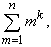Z. Anal. Anwendungen 18 (1999), no. 4, 1123-1130. In article View Article  J. Higgins, Double series for the Bernoulli and Euler numbers, J. London Math. Soc. 2nd Ser. 2 (1970), 722-726. In article View Article  S. Jeong, M.-S. Kim, and J.-W. Son, On explicit formulae for Bernoulli numbers and their counterparts in positive characteristic, J. Number Theory 113 (2005), no. 1, 53-68. In article View Article  F. Qi, Derivatives of tangent function and tangent numbers, Appl. Math. Comput. 268 (2015), 844-858. In article View Article  F. Qi and B.-N. Guo, Alternative proofs of a formula for Bernoulli numbers in terms of Stirling numbers, Analysis (Berlin) 34 (2014), no. 3, 311-317. In article View Article  S. Shirai and K.-I. Sato, Some identities involving Bernoulli and Stirling numbers, J. Number Theory 90 (2001), no. 1, 130-142. In article View Article  H.-F. Ge, New sharp bounds for the Bernoulli numbers and refinement of Becker-Stark inequalities, J. Appl. Math. 2012, Article ID 137507, 7 pages. In article View Article  C. D’Aniello, On some inequalities for the Bernoulli numbers, Rend. Circ. Mat. Palermo (2) 43 (1994), no. 3, 329-332. In article View Article  A. Laforgia, Inequalities for Bernoulli and Euler numbers, Boll. Un. Mat. Ital. A (5) 17 (1980), no. 1, 98-101. In article  D. J. Leeming, The real zeros of the Bernoulli polynomials, J. Approx. Theory 58 (1989), no. 2, 124-150. In article View Article  H. Alzer, Sharp bounds for the Bernoulli numbers, Arch. Math. (Basel) 74 (2000), no. 3, 207-211. In article View Article  Feng Qi, A double inequality for the ratio of two non-zero neighbouring Bernoulli numbers, Journal of Computational and Applied Mathematics 351 (2019), 1-5. In article View Article  Q.-M. Luo, B.-N. Guo, and F. Qi, On evaluation of Riemann zeta function ζ(s), Adv. Stud. Contemp. Math. (Kyungshang) 7 (2003), no. 2, 135-144. In article  Q.-M. Luo, Z.-L. Wei, and F. Qi, Lower and upper bounds of ζ(3), Adv. Stud. Contemp. Math. (Kyungshang) 6 (2003), no. 1, 47-51. In article  L. Yin and F. Qi, Several series identities involving the Catalan numbers, Trans. A. Razmadze Math. Inst. 172 (2018), no. 3, 466-474. In article View Article  B.-N. Guo, I. Mezö, and F. Qi, An explicit formula for the Bernoulli polynomials in terms of the r-Stirling numbers of the second kind, Rocky Mountain J. Math. 46 (2016), no. 6, 1919-1923 In article View Article  H.-L. Lv, Z.-H. Yang, T.-Q. Luo, and S.-Z. Zheng, Sharp inequalities for tangent function with applications, J. Inequal. Appl. 2017, Paper No. 94, 17 pp. In article View Article  F. Qi and R. J. Chapman, Two closed forms for the Bernoulli polynomials, J. Number Theory 159 (2016), 89-100. In article View Article  Z.-H. Yang, Approximations for certain hyperbolic functions by partial sums of their Taylor series and completely monotonic functions related to gamma function, J. Math. Anal. Appl. 441 (2016), no. 2, 549-564. In article View Article  L. Zhu, New bounds for the exponential function with cotangent, J. Inequal. Appl. (2018), 2018:106, 13 pages. In article View Article  L. Zhu, On Frame’s inequalities, J. Inequal. Appl. (2018), 2018:94, 14 pages. In article View Article  L. Zhu, Sharp generalized Papenfuss–Bach-type inequality, J. Nonlinear Sci. Appl. 11 (2018), no. 6, 770-777. In article View Article  L. Zhu and M. Nenezi´c, New approximation inequalities for circu- lar functions, J. Inequal. Appl. (2018). In article View Article  F. Qi, A double inequality for ratios of the Bernoulli numbers, ResearchGate Dataset. In article View Article  F. Qi, A double inequality for ratios of Bernoulli numbers, ResearchGate Dataset. In article View Article  F. Qi, A double inequality for ratios of Bernoulli numbers, RGMIA Res. Rep. Coll. 17 (2014), Article 103, 4 pages. In article View Article  F. Qi, A double inequality for the ratio of two consecutive Bernoulli numbers, Preprints 2017, 2017080099, 7 pages. In article View ArticleThis work is licensed under a Creative Commons Attribution 4.0 International License. To view a copy of this license, visit http://creativecommons.org/licenses/by/4.0/